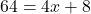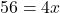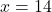Question

If the measure of Angle F G H is 64 degrees and the measure of Angle E G D is (4 x + 8) degrees, what is the value of x?

Lines E H and D F intersect at point G.

14

18

64

116

1.mocmien2

x = 14

Step-by-step explanation:

you should start by drawing a picture

when you do this, you will see that angle fgh and angle egd are vertical angles, that means they are congruent

therefore:2.If the measure of Angle F G H is 64 degrees and the measure of Angle E G D is (4 x + 8) degrees, what is the value of x?

Lines E H and D F intersect at point G.

14

18

64

116

Step-by-step explanation: